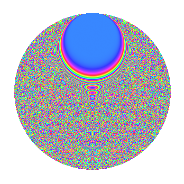# Properties

 Label 2541.2.bpLevel 2541 Weight 2 Character orbit bp Rep. character $$\chi_{2541}(64,\cdot)$$ Character field $$\Q(\zeta_{55})$$ Dimension 5280 Sturm bound 704

# Related objects

## Defining parameters

 Level: $$N$$ = $$2541 = 3 \cdot 7 \cdot 11^{2}$$ Weight: $$k$$ = $$2$$ Character orbit: $$[\chi]$$ = 2541.bp (of order $$55$$ and degree $$40$$) Character conductor: $$\operatorname{cond}(\chi)$$ = $$121$$ Character field: $$\Q(\zeta_{55})$$ Sturm bound: $$704$$

## Dimensions

The following table gives the dimensions of various subspaces of $$M_{2}(2541, [\chi])$$.

Total New Old
Modular forms 14240 5280 8960
Cusp forms 13920 5280 8640
Eisenstein series 320 0 320

## Trace form

 $$5280q - 4q^{2} + 128q^{4} - 4q^{7} + 8q^{8} - 1320q^{9} + O(q^{10})$$ $$5280q - 4q^{2} + 128q^{4} - 4q^{7} + 8q^{8} - 1320q^{9} - 16q^{10} + 12q^{11} - 16q^{12} + 92q^{13} + 6q^{14} - 8q^{15} + 164q^{16} - 8q^{17} + 6q^{18} - 8q^{19} + 60q^{20} + 16q^{21} + 8q^{22} + 28q^{23} + 156q^{25} + 60q^{26} - 2q^{28} - 16q^{29} + 8q^{30} + 32q^{31} - 88q^{32} - 8q^{33} - 56q^{34} + 128q^{36} + 28q^{37} + 4q^{38} - 32q^{39} - 4q^{40} - 8q^{41} + 48q^{43} + 4q^{44} + 6q^{46} + 16q^{47} + 16q^{48} + 132q^{49} + 592q^{50} - 24q^{51} + 244q^{52} + 52q^{53} + 176q^{55} + 118q^{56} + 8q^{57} + 88q^{58} + 4q^{59} + 48q^{60} + 188q^{62} - 4q^{63} + 212q^{64} + 92q^{65} + 52q^{67} - 88q^{68} + 12q^{69} + 36q^{70} + 180q^{71} - 2q^{72} + 36q^{73} + 28q^{74} + 32q^{75} + 236q^{76} - 16q^{77} + 180q^{78} + 140q^{79} - 136q^{80} - 1320q^{81} + 124q^{82} + 88q^{83} - 12q^{84} + 100q^{85} - 54q^{86} - 40q^{87} - 40q^{88} - 32q^{89} + 24q^{90} + 54q^{92} + 20q^{93} + 372q^{94} + 128q^{95} + 60q^{96} - 4q^{97} + 18q^{98} + 12q^{99} + O(q^{100})$$

## Decomposition of $$S_{2}^{\mathrm{new}}(2541, [\chi])$$ into newform subspaces

The newforms in this space have not yet been added to the LMFDB.

## Decomposition of $$S_{2}^{\mathrm{old}}(2541, [\chi])$$ into lower level spaces

$$S_{2}^{\mathrm{old}}(2541, [\chi]) \cong$$ $$S_{2}^{\mathrm{new}}(121, [\chi])$$$$^{\oplus 4}$$$$\oplus$$$$S_{2}^{\mathrm{new}}(363, [\chi])$$$$^{\oplus 2}$$$$\oplus$$$$S_{2}^{\mathrm{new}}(847, [\chi])$$$$^{\oplus 2}$$

## Hecke Characteristic Polynomials

There are no characteristic polynomials of Hecke operators in the database